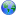Last update October 20, 2006

# How To / CGI

Difference (last change) (no other diffs, normal page display)

Changed: 1c1,78
 Describe the new page here.
 Using D in a CGI Environment I usually want my programs to be able to run in a web browser, ie you navigate an address to run the program and to use the arguments for execution. For example [[code]http://localhost/cgi-bin/MyDProgram?page=12345&something=else&another=keepitup] To do this we use the std.c.stdlib library (borrowed from C) Some useful functions: [[code] static import std.c.stdlib; static import std.string; char[] queryString() { return std.string.toString(std.c.stdlib.getenv("{QUERY STRING}?")); } ] This function simply retrieved the Query String (in the example: "page=12345&something=else&another=keepitup") If you want to use it as a module, you could use the following functions to give yourself a global _GET array hash map as you have available in PHP: [[code] module jcgi; // static makes the std.c.stdlib REQUIRED static import std.c.stdlib; static import std.string; // global variable char[][char[]] _GET; // called when module is imported, initializes the hash map (_GET) static this() { // Output content type jcgi.print("Content-type: text/html\n\n"); // Initialize _GET array. _GET = jcgi.set_GET(); } // fetch query string char[] queryString() { return std.string.toString(std.c.stdlib.getenv("{QUERY STRING}?")); } // assign values to _GET hash map (associative array) char[][char[]] set_GET() { char[][char[]] ret; char[] q = std.string.strip(jcgi.queryString()); char[][] args = std.string.split(q, "&"); delete q; for(int i = 0; i < args.length; i++) { char[][] tmp = std.string.split(args[i], "="); ret[tmp] = tmp; delete tmp; } delete args; return ret; } ] If you were hoping to have some more functions that PHP does (for testing purposes or whatever), you could use the following simple ones: [[code] static import io = std.stdio; void print_r(char[][char[]] arr) { foreach(key, value; arr) io.writefln("%s => %s", key, value); } void print(char[] string) { io.writefln(string); } ] I am wondering if anyone has a function written that could take a string like "hello \$name!" and turn it into "hello " ~ name ~ "!". Here is some simple stuff to get you started in using your D program in a web environment.

Using D in a CGI Environment
I usually want my programs to be able to run in a web browser, ie you navigate an address to run the program and to use the arguments for execution. For example`http://localhost/cgi-bin/MyDProgram?page=12345&something=else&another=keepitup`

To do this we use the std.c.stdlib library (borrowed from C) Some useful functions:```static import std.c.stdlib; static import std.string; char[] queryString() { return std.string.toString(std.c.stdlib.getenv("QUERY_STRING")); }```

This function simply retrieved the Query String (in the example: "page=12345&something=else&another=keepitup")

If you want to use it as a module, you could use the following functions to give yourself a global _GET array hash map as you have available in PHP:```module jcgi; // static makes the std.c.stdlib REQUIRED static import std.c.stdlib; static import std.string; // global variable char[][char[]] _GET; // called when module is imported, initializes the hash map (_GET) static this() { // Output content type jcgi.print("Content-type: text/html\n\n"); // Initialize _GET array. _GET = jcgi.set_GET(); } // fetch query string char[] queryString() { return std.string.toString(std.c.stdlib.getenv("QUERY_STRING")); } // assign values to _GET hash map (associative array) char[][char[]] set_GET() { char[][char[]] ret; char[] q = std.string.strip(jcgi.queryString()); char[][] args = std.string.split(q, "&"); delete q; for(int i = 0; i < args.length; i++) { char[][] tmp = std.string.split(args[i], "="); ret[tmp] = tmp; delete tmp; } delete args; return ret; }```

If you were hoping to have some more functions that PHP does (for testing purposes or whatever), you could use the following simple ones:```static import io = std.stdio; void print_r(char[][char[]] arr) { foreach(key, value; arr) io.writefln("%s => %s", key, value); } void print(char[] string) { io.writefln(string); }```

I am wondering if anyone has a function written that could take a string like "hello \$name!" and turn it into "hello " ~ name ~ "!".

Here is some simple stuff to get you started in using your D program in a web environment.

| | | | | | | | | |

(date of last change: October 20, 2006 2:59 )